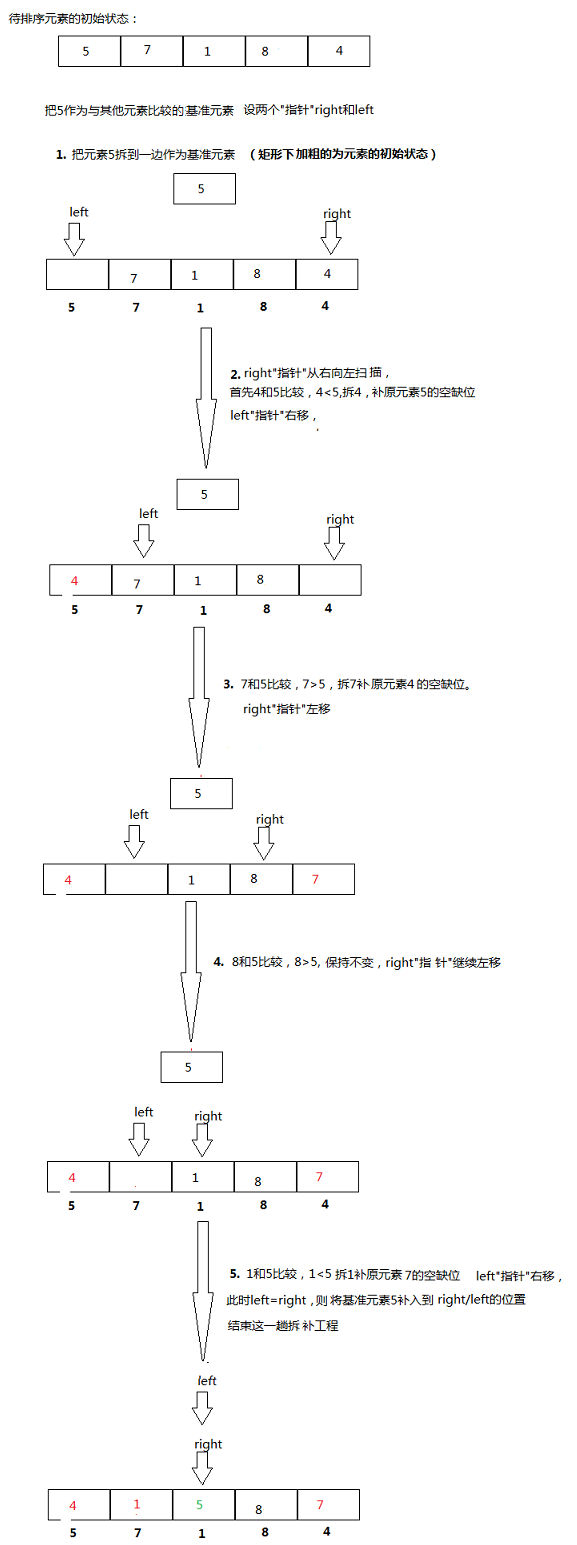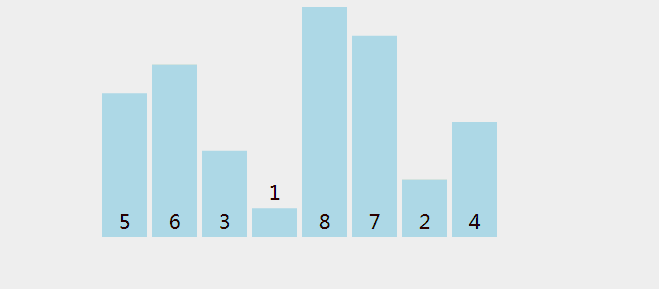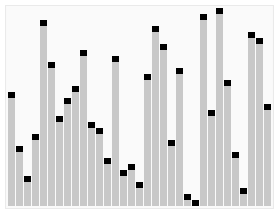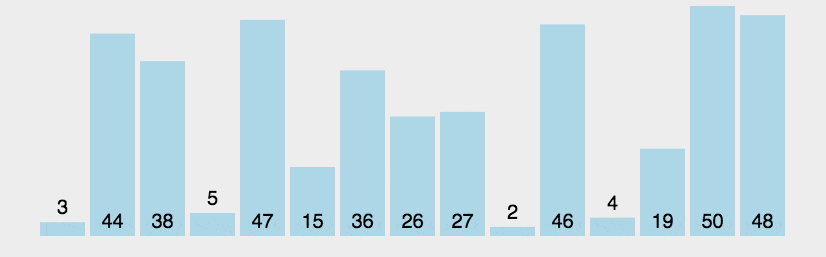•5星
469KB weixin_46414659 2021-04-02 17:24:37
•5星
852KB fenglingxicao 2021-01-10 21:24:50
•5星
19KB m0_52957036 2020-11-09 01:05:56
•5星
29KB weixin_44412576 2021-04-11 15:51:24
•5星
296KB m0_52957036 2020-09-06 23:56:19
•5星
387KB weixin_44510615 2021-06-23 18:04:15
•5星
14.4MB qq_37740841 2021-08-07 21:45:39
•5星
147KB weixin_45709829 2021-07-12 16:46:57
•5星
27KB qq_52889967 2021-07-08 19:52:37
•5星
11.24MB www_vane 2021-05-24 17:26:32
• 排序——交换类排序（冒泡排序、快速排序） Java 数据结构与算法 交换类排序 冒泡排序 快速排序

冒泡排序和快速排序就是典型的交换类排序算法。   2. 冒泡排序 冒泡排序也叫做相邻比逆，即在扫描待排序元素序列时，顺次比较相邻两个元素的大小，如果逆序就交换位置。 2.1 排序步骤...

1.  交换类排序算法的基本思想:

对待排序记录的关键字两两进行比较，只要发现两个记录为逆序就进行交换，直到没有逆序的记录为止。

如果要将整个记录序列调整力递增序列，那么元素之间是递减关系即为逆序。

冒泡排序和快速排序就是典型的交换类排序算法。

2.  冒泡排序

冒泡排序也叫做相邻比逆法，即在扫描待排序元素序列时，顺次比较相邻两个元素的大小，如果逆序就交换位置。

2.1 排序步骤

• 第 1 趟比较第 1 和第 2 个元素，如果逆序就交换，再依次比较第 2 和第 3 个、第 3 和第 4  ...  若是逆序则交换。经过该趟比较和交换，最大的数必然 “沉到” 最后一个元素。
• 第 2 趟用同样的方法，在前面的 n-1 个记录中，依次进行比较和交换，第 2 大的数“沉到”倒数第 2 个元素中。
• 第 i 趟仍用同样方法，在剩下的 n-i+1 个记录中，依次进行比较和交换，第 i 大的数“沉到”倒数第 i 个记录中。
• 重复此过程，直到 i=n-1 最后趟比较完为止。

【注】若在某一趟冒泡排序过程中，没有发现一个逆序，就可以直接结束整个排序过程。

package com.zth.sort;

import java.util.Arrays;

/**
* @author 时光·漫步
* 冒泡排序
*/
public class BubbleSort {
public static void main(String[] args){
Integer [] array1 = {3,2,5,8,4,7,6,9};
bubbleSort(array1);

Double[] array2 = {3.3,2.3,5.3,8.3,4.3,7.3,6.3,9.3};
bubbleSort(array2);

System.out.println(Arrays.toString(array1));
System.out.println(Arrays.toString(array2));

}

private static <AnyType extends Comparable<? super AnyType>>
void bubbleSort (AnyType[] array){
boolean flag = true;
AnyType temp;
// 遍历的趟数
for (int i = 0; (i < array.length - 1) && flag; i++) {
flag = false;
for (int j = 0; j < array.length - i-1; j++) {
if (array[j].compareTo(array[j+1])>0){
temp = array[j];
array[j] = array[j+1];
array[j+1] = temp;
flag = true;
}
}
}
}
}

2.2  性能分析

 时间复杂度 空间复杂度 稳定性 平均情况 最坏情况 最好情况 o(n^2) o(n^2) o(n) o(1) 稳定

3.  快速排序

快速排序采用分治的策略。

分治法的基本思想是：将原问题分解为若干个规模更小但结构与原问题相似的子问题，递归地解这些子问题，然后将这些子问题的解组合为原问题的解。

快速排序的基本思想为：从待排序列中任意选择一个元素， 以该元素作为枢纽元，小于枢纽元的元素均移动至该记录之前，反之，大于枢纽元的元素均移动至该记录之后。致使一趟排序之后，记录的无序序列  r[ 1... n ]  将分割成左右两个子序列，然后分别对分割所得两个子序列递归地进行快速排序，以此类推，直至每个子序列中只含一个记录为止。

3.1   枢纽元的选取

1.   选择第一个元素作为枢纽元。若果待排序元素是预排序的或反序的，性能将很差。

2.  随机选取枢纽元。虽然这是一种安全的做法，但随机数的生成开销较大。

3.  三数中值分隔法（常用）。使用左端、右端和中间位置的三个元素的中值作为枢纽元。

3.2  快排过程package com.zth.sort;

import java.util.Arrays;

public class  MyQuickSort{
public static void main(String[] args){

int[] array = {3,2,5,8,4,7,6,9};
quickSort(array,0,array.length-1);
System.out.println(Arrays.toString(array));
}

private static void quickSort (int[] array, int left,int right){

// i、j 记录本趟排序位置的起始和终止点
int i = left;
int j = right;
int pivot  = array[left];

while (left < right){
// 从右往左遍历
while (left < right && array[right] >= pivot )
right--;
// 找到比 pivot 小的元素
array[left] = array[right];

// 从左往右遍历
while (left < right && array[left] <= pivot )
left++;
// 找到比 pivot 大的元素
array[right] = array[left];
}
// 填入枢纽值
array[right] = pivot;
// 至少包含两个待排元素
if (right-1 >i) quickSort(array,i,right-1);
if (right+1 < j) quickSort(array,right+1,j);
}

}

缺点：  传统基准值的选定时每次参照顺序表的第一个元素作为基准值, 但是如果数组为有序的情况下，会大大降低排序效率，退化成冒泡排序时间复杂度升为O(n^2) 。

优化方案：

• 三数中值分隔法。
• 当待排序序列的长度分割到一定大小后，使用插入排序。对于很小且部分有序的数组，快排不如插排好。

代码实现：

package com.zth.sort;

import java.util.Arrays;

/**
* @author 时光·漫步
*/
public class QuickSort {
public static int CUTOFF = 5;   // CUTOFF 一般取 10
public static void main(String[] args){
Integer [] array = {3,2,5,8,4,7,6,9};
quickSort(array);
System.out.println(Arrays.toString(array));
}

/**
* 快排的驱动程序
*/
public static <AnyType extends Comparable<? super AnyType>>
void quickSort( AnyType[] array){
quickSort(array,0,array.length-1);
}

private static <AnyType extends Comparable<? super AnyType>> void
quickSort (AnyType[] array,int left,int right){

if (left + CUTOFF < right ){

// 获取枢纽元的值
AnyType pivot = median3(array,left ,right);
/**
* 如果不加 CUOFF 当只含有两个元素时，通过获取 pivot 已经排序完成
* 如果继续排序则会越界（从 left 开始向左遍历)
*/
if (left+1 == right){
return;
}
// 开始分区
int i = left,j = right-1;

for(; ;){
// 因为将 pivot 放在了 right-1 ，所以先从左往右搜索
// 因为获取 pivot 时已在最左、最有分别放了小于、大于 pivot 的元素，所以不会越界

while (array[++i].compareTo(pivot) <0 ){}
while (array[--j].compareTo(pivot) >0 ) {}

if (i < j){
swapReferences(array,i,j);
}else
break;
}

// 填回枢纽元
swapReferences(array,i,right-1);

if (left < i) quickSort(array,left,i-1);
if (i < right) quickSort(array,i+1,right);
}
else
insertSort(array,left,right);
}

/**
* return median of left,center and right
* @return 枢纽元的值
* 选取枢纽元的同时将三个元素中的最小值放在 array[left] 、最大值放在 array[right]
*/
private static <AnyType extends Comparable<? super AnyType>> AnyType
median3 (AnyType[] array,int left,int right){

int center = (left+right)/2;

if (array[center].compareTo(array[left]) <0)
swapReferences(array,left,center);
if (array[right].compareTo(array[left]) < 0)
swapReferences(array,left,right);
if (array[right].compareTo(array[center]) < 0)
swapReferences(array,right,center);

// 将枢纽元放在 right - 1
swapReferences(array,center,right-1);

return array[right-1];

}

private static <AnyType extends Comparable<? super AnyType>>
void swapReferences(AnyType[] array,int left,int right){
AnyType temp = array[left];
array[left] = array[right];
array[right] = temp;
}
private static <AnyType extends Comparable<? super AnyType>>
void insertSort(AnyType[] array ,int left,int right){

AnyType temp;
int j;

for (int i = left+1; i <= right; i++) {
temp = array[i];
for (j = i-1; j >=left && temp.compareTo(array[j])< 0 ; j--) {
array[j+1] = array[j];
}
array[j+1] = temp;
}
}
}

3.3  性能分析

 时间复杂度 空间复杂度 稳定性 平均情况 最坏情况 最好情况 O(n log n) O(n^2) O(n log n) O( log n) 不稳定

展开全文qq_41573234 2018-12-21 12:40:51
• 38KB weixin_38657848 2020-09-03 10:33:46
• 22KB weixin_38631729 2021-01-20 00:49:03
• 排序算法之交换类排序 排序 c语言

常见的两种交换类排序冒泡排序和快速排序。 冒泡排序（Bubble sort） 冒泡排序作为最基础的排序算法，它的排序思想也如其名，通过比较两个相邻数据的大小，来决定是否交换它们的位置，最后经过多轮排序最终是...

交换类排序

交换类排序的思想，顾名思义，就是在每一轮的排序过程，通过不断的交换来使每个元素到达最终的位置。常见的两种交换类排序有冒泡排序快速排序

冒泡排序（Bubble sort）

冒泡排序作为最基础的排序算法，它的排序思想也如其名，通过比较两个相邻数据的大小，来决定是否交换它们的位置，最后经过多轮排序最终是整个序列有序。

网上找到的gif动图：代码：

void sortBubble(int a[], int n){
int i=0, j=0;
int temp;
int flag;
for(i=0; i<n; i++){
flag = 0;
for(j=i+1;j<n;j++){
if (a[i]>a[j]){
temp = a[i];
a[i] = a[j];
a[j] = temp;
flag = 1;
}
}
if(flag == 0){
return;
}
}
}

int main()
{
int a[] = {1,2,3,2,5,6};
int i = 0;
sortPao(a, 6);
for(i = 0; i<6;i++){
printf("%d ",a[i]);
}
return 0;
}

分析：

从代码中可以看出最内层的交换操作可以看做是基本操作。

注意排序的结束条件是flag==0，就是在一趟排序中没有发生交换，所以冒泡排序的排序趟数跟原始序列有关

时间复杂度：当内层if语句始终成立时，也就是序列正好是逆序时，需要执行n(n-1)/2次，所以时间复杂度是

当内层if语句不执行时，也就是序列是是有序的时候，也就是需要遍历一次，时间复杂度是

所以综合上，平均时间复杂度就是

空间复杂度：从代码中就可以看出，整个排序过程只需要一个temp变量来辅助，所以空间复杂度是

快速排序(Quick sort)

快速排序也是一种交换类的排序算法，它的思想就是每一轮先选择当前序列中的一个关键字（通常是序列中的第一个）作为枢纽，然后从序列中从前往后找到一个比它大（小）的数据，再从后往前找到一个比它小（大）的数据，然后交换它们的位置，直到它们相遇，相遇的位置即是枢纽的位置，同时也将枢纽左边都是比它小（大）的，右边都是比它大（小）的，然后再通过递归来对子序列进行排序。

gif动图：代码：

void sortQuick(int a[], int low, int high) {
int i, j;
int pivot, temp;
center = a[low];
if(low < high) {
i = low;
j = high;
while(i < j) {
while(j > i && a[j] >= pivot) {   //from right to left to find one bigger than pivot
j--;
}
while(i < j && a[i] <= pivot) {   //from left to right to find one smaller than pivot
i++;
}
if(i<j) {                        //change position
temp = a[j];
a[j] = a[i];
a[i] = temp;
}else{
a[low] = a[i];
a[i] = pivot;
}
}
sortQuick(a, i+1, high);              //recur the process
sortQuick(a, low, i-1);
}
}

int main() {
int a[] = {1,2,3,2,5,6};
int i = 0;
sortQuick(a, 0, 5);
for(i = 0; i<6; i++) {
printf("%d ",a[i]);
}
return 0;
}

分析：

时间复杂度：快速排序最好情况（每次划分都是均匀划分）的时间复杂度是

最坏的情况（待排序的序列是正序或者逆序时），复杂度是

平均时间复杂度就是

对时间复杂度的具体分析以后填。

空间复杂度：从代码中可以看出，快排是通过递归实现的，需要栈的辅助，空间复杂度是

另外，快排的排序数和初始序列有关，序列越无序，效率越高；越接近无序，效率越低

ref:《2019数据结构高分笔记》（天勤版本）

展开全文FrankieHello 2018-10-19 21:58:42
• 交换类排序（冒泡排序及快速排序） 排序算法

交换类排序算法的基本思想是：对带排序记录的关键字两两比较，只要发现两个记录为逆序就进行交换，直到没有逆序为止 一.冒泡排序 冒牌排序也叫相邻比较，即在扫描带排序记录序列是，顺次比较两个记录的关键字...

交换类排序算法的基本思想是：对带排序记录的关键字两两比较，只要发现两个记录为逆序就进行交换，直到没有逆序为止
一.冒泡排序
冒牌排序也叫相邻比较法，即在扫描带排序记录序列是，顺次比较两个记录的关键字大小，如果逆序就交换记录
动画示意（此处用http://blog.csdn.net/wall1999/article/details/55192744中的动图）//这里是c语言的实现，js也可以用，思想相同,稍微改动即可
void BubbleSort(RecordList L){
flag=1;
for(i=0;i<=L.length-1&&flag;i++)//只有flag为1才排序
{
flag=0;
for(j=0;j<L.length-i;j++)//注意此处为length-i，前面排过序的不必在排，去掉他们
{
if(L.r[j].key>l.r[j+1].key)//如果前一个数大于后面的数就交换位置，因此此处是升序排序
{
t=L.r[j];
L.r[j]=L.r[j+1];
L.r[j+1]=t;
flag=1;
}
}
}
}


由上述代码可以看出，如果不需要排序，则flag不会由0改为1，因此外层循环判断不成立，不再排序。
**时间复杂度：最好的情况下已经为正序，那么外层循环只进行1次就结束整个过程，最小时间代价为O(n)，但最差情况下，外层循环要进行n-1次，每一次外层循环控制内层循环进行n-1次，所以为O(n^2)**

二.快速排序
数据结构中描述快速排序的基本思想为：从带排序序列中任选一个作为‘枢轴’，小于枢轴的移动在枢轴前，大于的移动到枢轴后，一趟排序后无序序列被分为左右两个子序列，然后分别对分隔所得的子序列递归地进行快速排序，以此类推，直到每个子序列只含有一个记录为止。

function QKSort(myArr){
// 如果只有一位，就没有必要比较
if(myArr.length<=1){
return myArr;
}
// 获取中间值的索引
var len = Math.floor(myArr.length/2);
// 截取中间值
var cur = myArr.splice(len,1);//从中间值的位置删除一项，即把中间值从原数组中提出来，并复制给cur，因为splice会返回一个包含被删除项的数组
// 小于中间值放这里面
var left = [];
// 大于的放着里面
var right = [];
for(var i=0;i<myArr.length;i++){
// 判断是否大于
if(cur>myArr[i]){
left.push(myArr[i]);
}else{
right.push(myArr[i]);
}
}

// 通过递归，上一轮比较好的数组合并，并且再次进行比较。
return QKSort(left).concat(cur,QKSort(right));

}
展开全文qq_33276399 2017-07-25 09:28:03
• C++实现交换排序算法（冒泡排序、快速排序） 数据结构 排序算法 快速排序

交换类：冒泡排序、快速排序 选择：简单选择排序、堆排序 归并：二路归并排序 基数：多关键字排序 九种算法的时间复杂度、空间复杂度和稳定性小结如下： 本文放出选择算法的两种排序算法代码。 冒泡排序 void...

排序算法分为五大类，一共是有九种，如下：
插入类：直接插入排序、折半插入排序、希尔排序
交换类：冒泡排序、快速排序
选择类：简单选择排序、堆排序
归并类：二路归并排序
基数类：多关键字排序
九种算法的时间复杂度、空间复杂度和稳定性小结如下：本文放出交换算法的两种排序算法代码。
八种常用的排序算法代码可以到这里下载。

冒泡排序

void BubbleSort(int R[], int n) {
int i, j, temp, flag;
cout << endl << "冒泡排序：" << endl;
for (i = n - 1; i >= 1; --i) {
flag = 0;
for (j = 1; j <= i; ++j) {
if (R[j - 1] > R[j]) {
temp = R[j];
R[j] = R[j - 1];
R[j - 1] = temp;
flag = 1;
}

}
for (int k = 0; k < n; k++)
cout << R[k] << " ";
cout << endl;
if (flag == 0)
{
cout << endl;
return;
}
}
}

快速排序

void QuickSort(int R[], int low, int high) {
int temp, i = low, j = high;
if (low < high) {
temp = R[low];
while (i < j) {
while (j > i && R[j] >= temp)--j;
if (i < j) {
R[i] = R[j];
++i;
}
while (i < j && R[i] < temp)++i;
if (i < j) {
R[j] = R[i];
--j;
}
}
R[i] = temp;
for (int k = 0; k <=high; k++)
cout << R[k] << " ";
cout << endl;
QuickSort(R, low, i - 1);
QuickSort(R, i + 1, high);
}
}

总体

#include <iostream>
using namespace std;
void BubbleSort(int R[], int n) {
int i, j, temp, flag;
cout << endl << "冒泡排序：" << endl;
for (i = n - 1; i >= 1; --i) {
flag = 0;
for (j = 1; j <= i; ++j) {
if (R[j - 1] > R[j]) {
temp = R[j];
R[j] = R[j - 1];
R[j - 1] = temp;
flag = 1;
}

}
for (int k = 0; k < n; k++)
cout << R[k] << " ";
cout << endl;
if (flag == 0)
{
cout << endl;
return;
}
}
}
void QuickSort(int R[], int low, int high) {
int temp, i = low, j = high;
if (low < high) {
temp = R[low];
while (i < j) {
while (j > i && R[j] >= temp)--j;
if (i < j) {
R[i] = R[j];
++i;
}
while (i < j && R[i] < temp)++i;
if (i < j) {
R[j] = R[i];
--j;
}
}
R[i] = temp;
for (int k = 0; k <=high; k++)
cout << R[k] << " ";
cout << endl;
QuickSort(R, low, i - 1);
QuickSort(R, i + 1, high);
}
}
int main()
{
int A = { 49,38,65,97,76,13,27,49 };
int n = 8;
int R;
cout << "原始数组：";
for (int k = 0; k < n; k++) {
R[k] = A[k];
cout << R[k] << " ";
}
cout << endl;
BubbleSort(R, n); //冒泡排序
cout << "原始数组：";
for (int k = 0; k < n; k++) {
R[k] = A[k];
cout << R[k] << " ";
}
cout << endl;
int low = 0, high = 7;
cout << "快速排序" << endl;
QuickSort(R, low, high); //快速排序
cout << endl;
return 0;
}

主要参考的书籍是《数据结构高分笔记》2021版

展开全文qq_38222051 2020-11-01 22:10:44
• 排序算法(三)交换类排序:冒泡排序和快速排序 冒泡排序 快速排序

wenqiang1208 2017-08-03 23:25:50
• 排序算法 交换排序(冒泡排序、快速排序) 交换排序 排序算法 算法 交换排序算法 快速排序

qq_36511401 2019-10-19 14:44:58
• 交换类排序详解及总结 冒泡排序 快速排序 快速排序非递归

Number_0_0 2017-03-21 15:46:44
• 快速排序的交换次数 算法 数据结构 快速排序

weixin_44034024 2020-04-01 11:49:44
•5星
12KB xjm199 2013-11-04 14:23:16
• 排序算法——交换类排序 排序算法 冒泡排序 快速排序 算法

LaL15281183992 2016-12-11 00:52:16
• 排序（四）之快速排序 指针交换法 快速排序 快速排序指针交换法 java排序

u012326462 2018-09-11 21:16:34
• 510KB yanyanwenmeng 2017-12-04 21:28:27
• 两种常用的交换排序算法--冒泡排序、快速排序 快速排序 冒泡排序 交换排序

qq_35580883 2017-11-23 13:14:29
• qq_40941722 2019-07-01 17:27:49
• qq_36189382 2018-07-26 19:04:05
• 论文研究-在快速排序法中引入对相同数据的处理 .pdf 快速排序法

275KB weixin_39840914 2019-08-15 10:39:32
• yrk0556 2019-03-14 10:45:25
• 内部排序算法（一）：交换排序（冒泡排序，快速排序） 数据结构 排序 内排序算法 冒泡排序 快速排序

u013275340 2014-08-25 23:55:42
• 快速排序法（C语言实现） C语言 算法 快速排序

peng_apple 2018-03-28 11:51:03
• 常见的排序算法——交换排序 交换排序 快速排序 冒泡排序

LYJwonderful 2018-05-29 16:38:40
• 详谈排序算法之交换类排序（两种方法实现快速排序【思路一致】） 排序算法 快速排序 子集划分

qq_21394609 2015-02-23 00:18:24
• 六大排序算法：插入排序、希尔排序、选择排序、冒泡排序、堆排序、快速排序 数据结构 快速排序 排序算法 堆排序 插入排序

weixin_50886514 2021-07-23 21:56:09
• 436B lianyi237 2013-11-18 17:43:24
• 47KB weixin_38519849 2020-12-25 13:23:27
• C语言中数组的排序算法详解——选择法、冒泡法、交换法、插入法、折半法 算法 数据结构 排序算法 c语言

PGY_yyy 2020-06-16 19:41:53
• 排序算法之交换排序（冒泡排序、快速排序） 排序 数据结构

swpu_ocean 2018-11-12 13:52:24
• 快速排序的三种方法 快速排序 hoare

ych9527 2021-01-09 15:23:49...